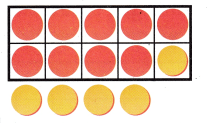Refer to our Texas Go Math Grade 1 Answer Key Pdf to score good marks in the exams. Test yourself by practicing the problems from Texas Go Math Grade 1 Lesson 6.6 Answer Key Make a 10 to Add.

Essential Question
How do you use the make a ten strategy to add?
Explanation:
6 + 5
Breaking 5 as 4 and 1
To make 10
6 + 4 = 10
10 + 1 = 11
6 + 5 = 11

Explore
What is 9 + 6? Use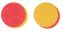and the ten frame. Model and draw to solve.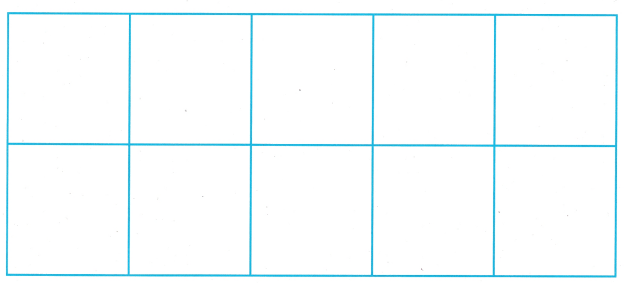For The Teacher

• Ask children: What is 9 + 6? Have children use red and yellow counters to model. Then move one counter from the 6 to make a ten.
Explanation:9 + 6
Breaking 6 as 5 and 1
9 + 1 = 10
10 + 5 = 15
9 + 6 = 15

Math Talk
Explain why you start by putting 9 counters in the ten frame.
Explanation:
Because, we have to add 1 to 9 to make 10
so that we can count on.

Model and Draw

Why do you show 8 in the ten frame to find 4 + 8?Explanation:
8 + 4
Breaking 4 as 2 and 2
8 + 2 = 10
10 + 2 = 12
8 + 4 = 12

Share and Show

Useand a ten frame. Show both addends. Draw to make a ten. Then write the new fact. Add.

Question 1.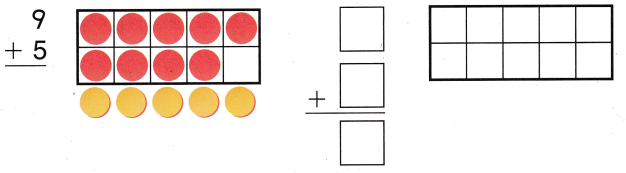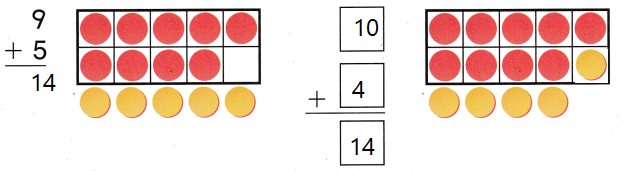Explanation:
9 + 5
Breaking 5 as 1 and 4
9 + 1 = 10
10 + 4 = 14
9 + 5 = 14

Question 2.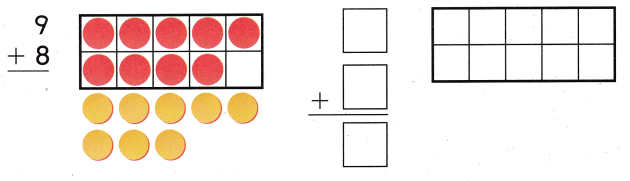Explanation:
9 + 8
breaking 8 as 1 and 7
9 + 1 = 10
10 + 8 = 18

Problem Solving

Use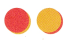and a ten frame. Show both addends. Draw to make a ten. Then write the new fact. Add

Question 3.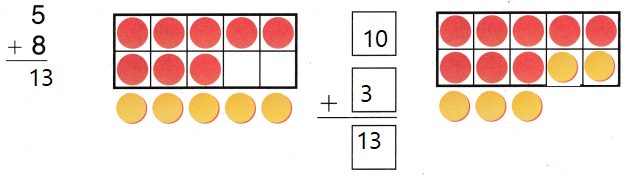Explanation:
5 + 8
breaking 5 as 2 and 3
8 + 2 = 10
10 + 3 = 13
5 + 8 = 13

Question 4.
H.O.T. Explain What strategy would you choose to solve 7 + 8? Why?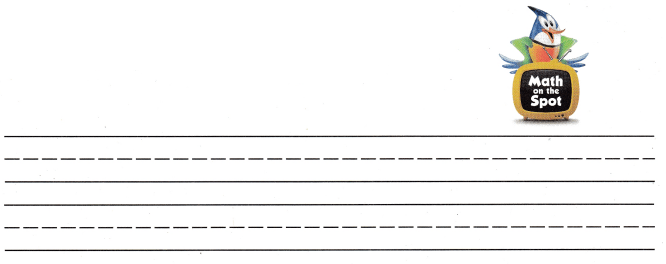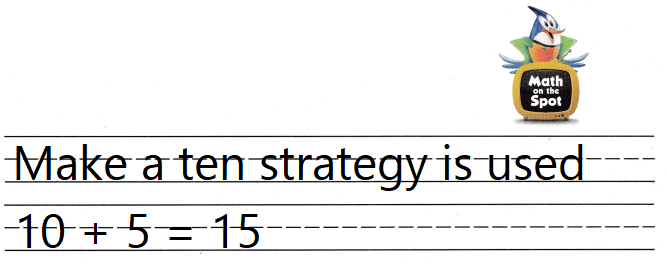Explanation:
7 + 8
Breaking 7 as 2 and 5
8 + 2 = 10
10 + 5 = 15

Draw to make a ten. Write the missing number.

Question 5.
H.O.T. Multi-Step Andrew has visited Cedar Hill State Park 7 times. Cathy has visited the same park 9 times. How many times did they both visit the park?
____ visits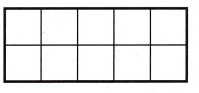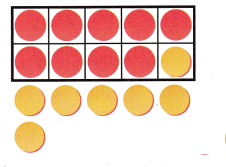Explanation:
Andrew has visited Cedar Hill State Park 7 times. Cathy has visited the same park 9 times.
7 + 9 = 16
7 as 1 and 6
9 + 1 = 10
10  + 6 = 16
16 times that they both visit the park

Question 6.
Apply A bird chirps 8 times. Then he chirps 6 more times. How many times does the bird chirp?
(A) 14
(B) 15
(C) 16Explanation:
A bird chirps 8 times. Then he chirps 6 more times.
8 + 6 = 14
14 times that the bird chirp

Question 7.
Kaitlyn wants to find 9 + 4. She makes a ten to add. Which addition fact does she use?
(A) 4 + 4 = 8
(B) 10 + 3 = 13
(C) 9 + 10 = 19
Explanation:
Kaitlyn wants to find 9 + 4. She makes a ten to add.
9+ 4
Breaking 4 as 1 and 3
9 + 1 = 10
10 + 3 = 13
9 + 4 = 13
13 is the addition fact that  she use

Question 8.
Luke has 5 crayons. He finds 8 more. How many crayons does Luke have now?
(A) 11
(B) 12
(C) 13Explanation:
Luke has 5 crayons. He finds 8 more.
5 + 8 = 13
13 crayons that Luke have now

Take Home Activity

• Cut off 2 cups from an egg carton, or draw a 5-by-2 grid on a sheet of paper to create a ten frame. Have your child use small objects to show how to make a ten to solve 8 + 3, 7 + 6. and 9 + 9.
Explanation:
8 + 3
3 as 2 and 1
8 + 2 = 10
10 + 1  = 11
Explanation:
7 + 6
6 as 3 and 3
7 + 3 = 10
10 + 3 = 13
Explanation:
9 + 9
9 as 1 and 8
9 + 1 = 10
10 + 8 = 18

### Texas Go Math Grade 1 Lesson 6.6 Homework and Practice Answer Key

Draw to make a ten. Then write the new fact. Add.

Question 1.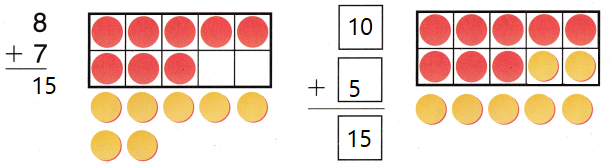Explanation:
8 + 7
Breaking 7 as 2 and 5
8 + 2 = 10
10 + 5 = 15
8 + 7 = 15

Question 2.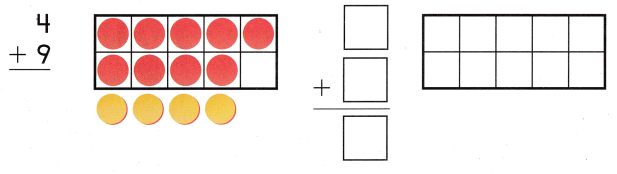Explanation:
4 + 9
Breaking 4 as 1 and 3
9 + 1 = 10
10 + 3 = 13

Problem Solving

Draw to make a ten. Write the missing number.

Question 3.
Jana got 5 new books. Lou got 7 new books. How many new books did Jana and Lou get?___ new books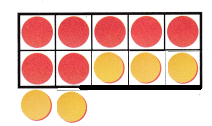Explanation:
Jana got 5 new books. Lou got 7 new books.
7 + 3 = 10
10 + 2 = 12
12 new books that Jana and Lou get

Texas Test prep

Question 4.
7 acorns fall from an oak tree. Then 9 more acorns fall from the tree. How many acorns fall from the tree?(A) 15
(B) 16
(C) 17
Explanation:
7 acorns fall from an oak tree. Then 9 more acorns fall from the tree.
9 + 7 = 16
16 acorns fall from the tree

Question 5.
A squirrel hides 6 acorns. Then he hides 8 more acorns. Which addition fact can you use to solve the problem?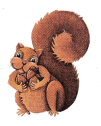(A) 10 + 4 = 14
(B) 8 + 10 = 18
(C) 10 + 6 = 16
Explanation:
A squirrel hides 6 acorns. Then he hides 8 more acorns.
6 + 8
Breaking 8 as 4 and 4
6 + 4 = 10
10 + 4 = 14
is the addition fact that can be use to solve the problem

Question 6.
Multi-Step Len’s mother uses 9 eggs. Then she uses 1 more egg and then 3 more eggs. How many eggs does Len’s mother use?
(A) 15
(B) 12
(C) 13
Explanation:
Len’s mother uses 9 eggs. Then she uses 1 more egg and then 3 more eggs.
9 + 1 = 10
10 + 3 = 13
13 eggs that Len’s mother use

Question 7.
What number sentence does this model show?
(A) 10 + 1 = 11
(B) 10 + 4 = 14
(C) 10 + 5 = 15